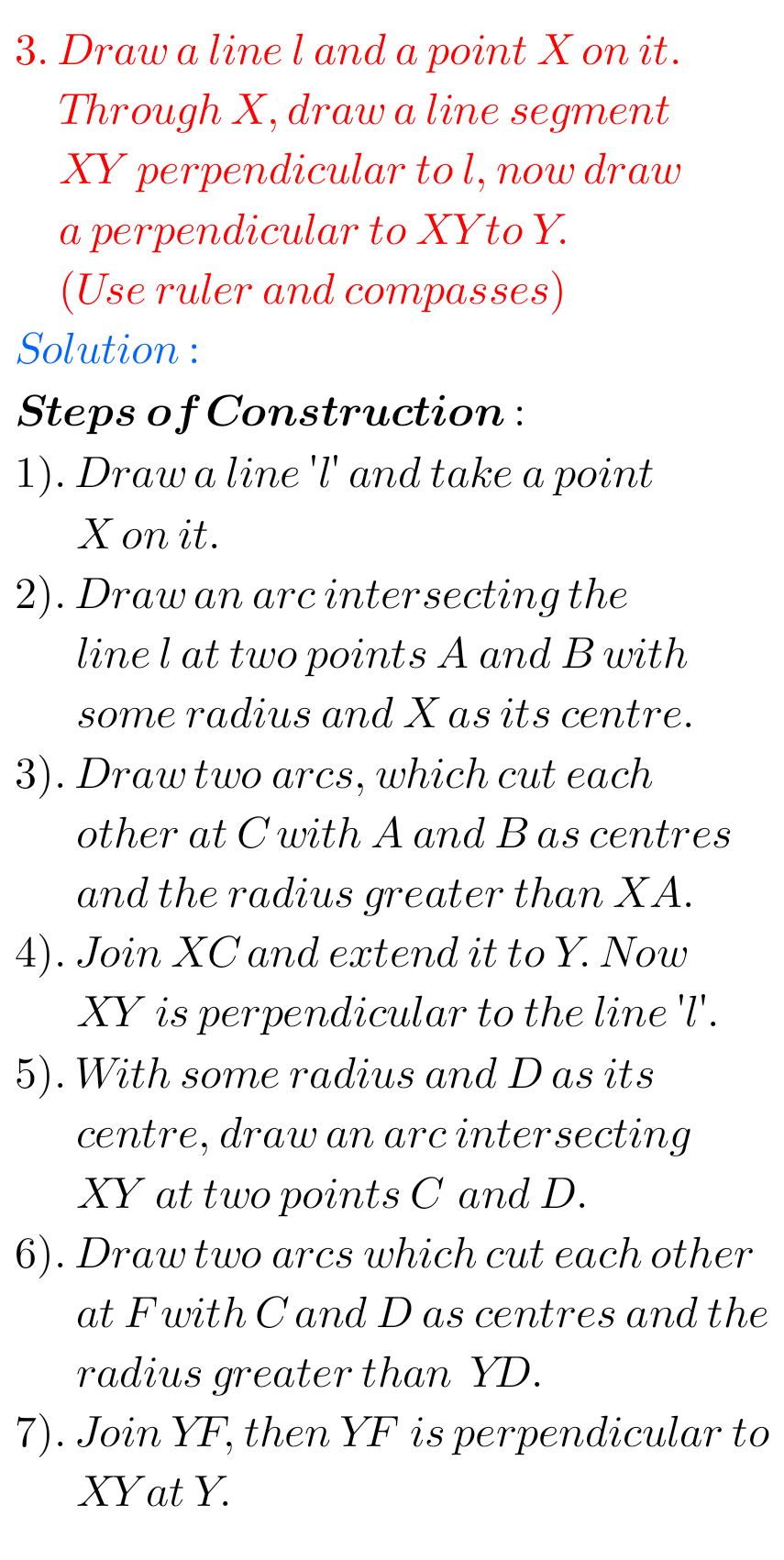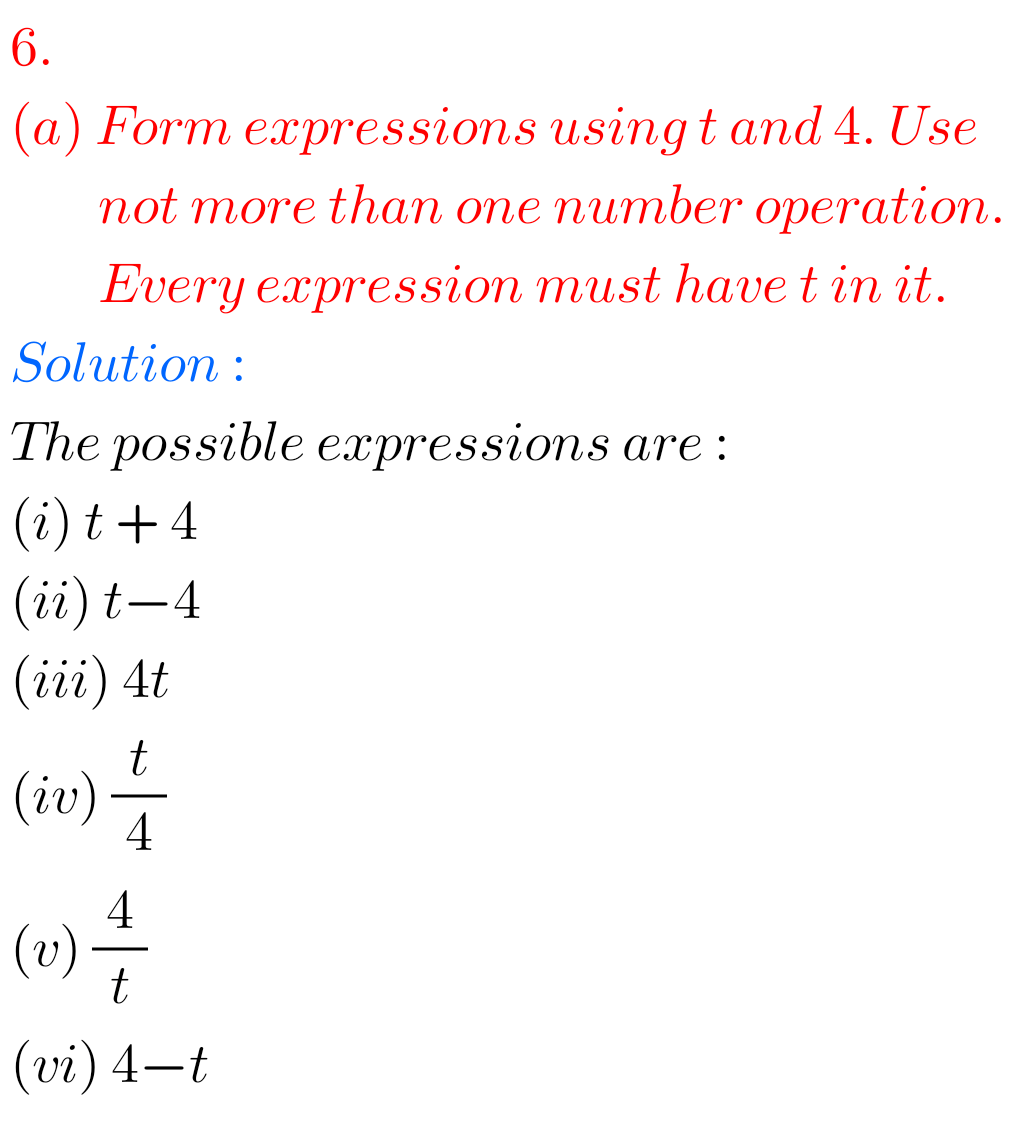# cbse maths solutions## Ncert maths solutions for class 6 Decimals chapter 8 Exercise 8.1

Decimals solutions class 6 ncert maths exercise 8.1 Maths Ncert Class 6 textbook Chapter 8 Decimals Exercise 8.1 solutions are given. First you should study the textbook lesson Decimals very well. You should observe the example problems and solutions given in the textbook. Observe the given solutions and try them in your own method. You …## Ncert solutions for class 6 Maths chapter 5 exercise 5.9

Exercise 5.9 solutions chapter 5 class 6 Maths Ncert Understanding Elementary Shapes Ncert Maths Class 6 chapter 5 Understanding Elementary Shapes Exercise 5.9 solutions are given. You should study the textbook lesson Understanding Elementary Shapes very well. You should observe the example problems and solutions given in the textbook. Observe the given solutions and try …

## NCERT solutions for class 7th maths chapter 2/Fractions and Decimals solutions

NCERT SOLUTIONS FOR CLASS 7TH MATHS CHAPTER 2 FRACTIONS AND DECIMALS Fractions and Decimals Chapter 2 class 7 Maths NCERT solutions are given. You should study the textbook lesson Fractions and Decimals very well. You should observe and practice the example problems and solutions given in the textbook. Observe the given below solutions and try …## Ncert solutions for class 7th maths chapter 4 Simple Equations, problems with solutions for Simple Equations vii class cbse

NCERT SOLUTIONS FOR CLASS 7 TH MATHS CHAPTER 4, SIMPLE EQUATIONS Simple Equations class 7-chapter 4 maths NCERT solutions are given. You should study the textbook lesson Simple Equations very well. You should observe and practice the example problems and solutions given in the textbook. Observe the given below solutions and try them in your …## Ncert Maths Chapter 14 Practical Geometry Exercise 14.4 Solutions Class 6

Practical Geometry Solutions Exercise 14.4 Class 6 Ncert Ncert solutions for Mathematics Class 6th textbook Chapter 14 Practical Geometry Exercise 14.4 are given. First you study the textbook lesson Practical Geometry very well. You should also observe the example problem and solutions given in the textbook. Observe the given below solutions and try them in …## Exercise 14.3 Practical Geometry solutions class 6 Ncert

Practical Geometry Solutions Class 6 Ncert Exercise 14.3 Ncert solutions for Mathematics Class 6th textbook Chapter 14 Practical Geometry Exercise 14.3 are given. First you should study the textbook lesson Practical Geometry very well. You should observe the example problem and solutions given in the textbook. Observe the given below solutions and try them in …## Practical Geometry Solutions exercise 14.2 Ncert Class 6 Maths

Ncert Solutions for Maths class 6 Practical Geometry Exercise 14.2 Ncert solutions for Mathematics Class 6th textbook Chapter 14 Practical Geometry Exercise 14.2 are given. You should study the textbook lesson Practical Geometry very well. You should also observe the example problems and solutions given in the textbook. Observe the given below solutions and try …## Ncert solutions for class 6 Practical Geometry Exercise 14.1

Practical Geometry Exercise 14.1 Solutions Ncert class 10 Practical Geometry Exercise 14.1 Solutions Ncert Class 6th textbook are given. First you should study the textbook lesson Practical Geometry very well. You should also observe the example problems and solutions given in the textbook. Observe the given below solutions and try them in your own method. …## Algebra solutions for exercise 11.3 class 6 Ncert Maths

Solutions for Algebra Exercise 11.3 Chapter 11 class 6 Ncert Maths Class 6-chapter 11 Algebra exercise 11.3 solutions Maths Ncert textbook are given. You should study the textbook lesson Algebra very well. You should also observe the example problems and solutions given in the textbook. Observe the solutions and try them in your own method. …## Solutions for Basic Geometrical Ideas Exercise 4.5 Maths Class 6 Ncert

Basic Geometrical Ideas Exercise 4.5 Solutions Class 6 Ncert Ncert textbook Mathematics Class 6 Chapter 4 Basic Geometrical Ideas exercise 4.5 solutions are given. Study the lesson Basic Geometrical Ideas in the textbook. Observe the problems and solutions given in the textbook. Observe the given below problems and solutions and try them in your own …